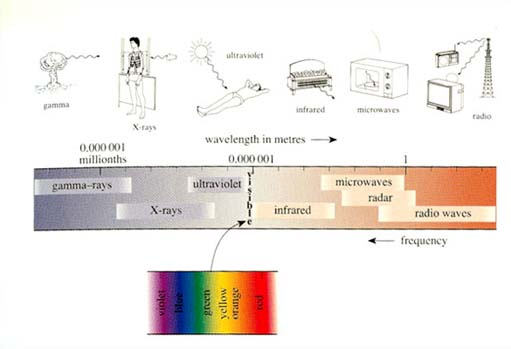The Sun

This free course is available to start right now. Review the full course description and key learning outcomes and create an account and enrol if you want a free statement of participation.

Free course

# 1.3 Beyond visible light

During the twentieth century, astronomers extended their capabilities by developing telescopes and detectors that were sensitive to radio waves, microwaves, infrared and ultraviolet radiation, X-rays and gamma rays. All these forms of electromagnetic radiation, along with visible light, are emitted by the Sun.Figure 6 The electromagnetic spectrum

This is a good place to examine the electromagnetic spectrum in more detail and to review some of its features. Figure 6 shows the wavelength values in metres but only some of the marks are labelled. The marks go up in 'times ten' steps so, to the right of the 1 metre mark, the first (unlabelled) mark indicates a wavelength of 10 metres (10 m), the next 100 m and the third 1000 m. Going to the left of the 1 metre mark, the next mark is 0.1 m, then 0.01 m (which is 1 centimetre or 1 cm for short), 0.001 m (1 millimetre or 1 mm), and so on. Going further to the left involves putting more and more zeroes after the decimal point, so the wavelengths are sometimes measured in micrometres - millionths of a metre. One micrometre is 0.000 001 metres.

## Question 2

• What is the definition of the wavelength of an electromagnetic wave?

It is the distance between successive peaks of the wave.

## Question 3

• Roughly, what is the wavelength of visible light?

It is about 0.000 001 metres (actually, 0.000 000 4 to 0.000 000 7 metres).

## Question 4

• What type of electromagnetic radiation has the longest wavelength? What, approximately, is its wavelength range?

Radio waves are the longest: in Figure 6, the shortest radio waves lie about one step to the left of the 1 m mark, which denotes a wavelength of 0.1 m. The band representing radio waves extends to the far right of the chart. The furthest mark on the right represents a wavelength of 1000 m (three steps to the right of the 1 m mark), so the figure shows that radio waves can have wavelengths of over 1 kilometre. (Such wavelengths are typical of radio stations still broadcasting in the 'long wave' band.)

S194_1